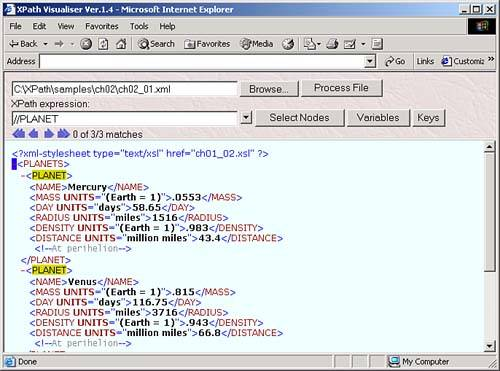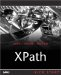Working with Node-Sets

Working with Node-Sets

As you know, node-sets are XPath's way of dealing with multiple nodes. For example, you can see the node-set returned by the expression //planet on our sample XML document in the XPath Visualiser in Figure 2.7. But there's more to know about node-sets.

Figure 2.7. A node-set.When you're working with a node-set, XPath gives you a variety of resources that are available at any time called the XPath context . You'll see more about what's in the XPath context in the upcoming chapters; here's what in it:

• The context node , which is the XML node in the XML document that the XPath expression was invoked on. In other words, XPath expressions are executed starting from the context node. We'll see how to use relative expressions in XPath soon, and such expressions are always relative to the context node.

• The context position , which is a nonzero positive integer indicating the position of a node in a node-set. The first node has position 1, the next position 2, and so on.

• The context size , which is also a nonzero positive integer, the context size gives the maximum possible value of the context position. (It's the same as the number of nodes in a node-set.)

• A set of variables you can use variables to hold data in XSLT, and if you do, those variables are stored in the expression's context, which can be accessed in XPath.

• A function library full of functions ready for you to call, such as the sum function, which returns the sum of the numbers you pass it.

• The set of XML namespace declarations available to the expression.

In addition to these context items, there is also the current node, which we've already discussed. The current node is not the same as the context node . The context node is set before you start evaluating an XPath expressionit's the node the expression is invoked on. However, as the XPath processor evaluates an XPath expression, it can work on various parts of that expression piece by piece, and the node that the XPath processor is working on at the moment is called the current node.

Here's an example showing how to work with context nodes and positions . Say that you apply the XPath expression /planets/planet to our planetary data:

<?xml version="1.0"?> <planets>     <planet>         <name>Mercury</name>         <mass units="(Earth = 1)">.0553</mass>         <day units="days">58.65</day>         <radius units="miles">1516</radius>         <density units="(Earth = 1)">.983</density>         <distance units="million miles">43.4</distance>         <!--At perihelion-->     </planet>     <planet>         <name>Venus</name>         <mass units="(Earth = 1)">.815</mass>         <day units="days">116.75</day>         <radius units="miles">3716</radius>         <density units="(Earth = 1)">.943</density>         <distance units="million miles">66.8</distance>         <!--At perihelion-->     </planet>         .         .         .

The first / in /planets/planet makes the root node the context node for the rest of the expression. The planets part makes the <planets> element the context node for the rest of the expression after that point. That means that the remainder of this expression, /planet , will be evaluated with respect to the <planets> element, so the <planets> element is the context node for the /planet part of this XPath expression.

The whole expression, /planets/planet , matches and returns the three <planet> elements in a node-set. The first <planet> element will have the context position 1, the next will have context position 2, and so on. The context size of the node-set containing the three <planet> elements is three.

Here's an example showing how to work with the variables present in a node-set context. XPath doesn't let you define variables. However, you can create variables in an XSLT stylesheet with the <xsl:variable> element like this, where I'm creating a variable named myPosition with the value 3:

<xsl:variable name="myPosition" select="3"/>

This new XSLT variable, myPosition , can be used in XPath expressions. For example, as we saw in Chapter 1, you can assign XPath expressions to the XSLT <xsl:value-of> element's select attribute. And in XPath, you can refer to the value in a variable by prefacing the variable's name with a \$ , as you see in ch02_02.xsl in Listing 2.2.

Listing 2.2 Using an XSLT Variable ( ch02_02.xsl )
<?xml version="1.0"?>  <xsl:stylesheet version="1.0" xmlns:xsl="http://www.w3.org/1999/XSL/Transform">     <xsl:template match="//planets">         <HTML>             <xsl:apply-templates/>         </HTML>     </xsl:template>     <xsl:variable name="myPosition" select="3"/>     <xsl:template match="planet">         <P>  <xsl:value-of select="\$myPosition"/>  </P>     </xsl:template> </xsl:stylesheet>

This will insert the value of myPosition into the document. This stylesheet just replaces each <planet> element with the value in myPosition , which is 3, in a <P> element, this way:

<HTML>     <P>     3     </P>     <P>     3     </P>     <P>     3     </P> </HTML>

And we've already seen some of the XPath functions, such as the position function, which we've used like this: //planet[position()=3] , where we're using the position() function to return the current node's context position. All the XPath 1.0 functions are coming up in Chapter 4.

String Value of Node-Sets

We've already seen that nodes have string values, and it turns out that node-sets also have string values in XPathbut a node-set's string value might surprise you. If you followed the discussion earlier about the string value of a root node, which is the concatenation of text nodes in the document, you might expect the string-value of a node-set to be made up of the concatenated string-values of all the nodes in the set.

But that's not soin XPath, the string-value of a node-set is simply the string-value of the first node in the node set only. For example, if you apply the XPath expression //planet to our planets example, ch02_01.xml , you'll get a node-set holding the three <planet> elements in that document, in document order. However, the string value of this node-set is the string value of the first element only, the Mercury element:

<planet>     <name>Mercury</name>     <mass units="(Earth = 1)">.0553</mass>     <day units="days">58.65</day>     <radius units="miles">1516</radius>     <density units="(Earth = 1)">.983</density>     <distance units="million miles">43.4</distance>     <!--At perihelion--> </planet>

Here's the string value of this element, and therefore of the entire //planets node-set:

Mercury .0553 58.65 1516 .983 43.4

That completes our look at nodes and node-sets. The next step up in organization in XPath is to start thinking in terms of node trees .XPath Kick Start: Navigating XML with XPath 1.0 and 2.0
ISBN: 0672324113
EAN: 2147483647
Year: 2002
Pages: 131

Similar book on Amazon